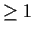Next: *timsort* Up: Timing Analysis Previous: Summary of tasks in

## An example of a typical timing analysis

As an example we use the Einstein Observation of CTB109, a supernova remnant with bright internal pulsar. Data are found in the PROS package directory ``xdata''. First the source events are extracted from the rest of the field using a circular region centered on the source. Events will be time ordered in the output file.

```  xt> timsort xdata\$snr psr ``c 503 513 24'' none
```
A light curve is then generated and plotted so we can inspect the gross properties of the sample (the sequence of good-data intervals and gaps) and any source variability.
```  xt>  ltcurv psr none psr 500

xt>  ltcplot psr_ltc
```
Finding this sample satisfactory, we search for periodicity with a fast Fourier transform. We use 214 bins so the search will cover periodss in this 7700 s data interval.
```  xt>  fft psr_sti.qp none psr src 16384
```
The result is viewed by plotting power as a function of frequency and by listing the table containing significant coefficients.
```  xt>  fftplot.y_range = "0 20"

xt>  fftplot psr_fft

xt>  timprint psr_pwr 1-20
```
We find significant power at low frequency because of the gaps in the data record and, at a frequency of 0.2868-1, the pulsar. The period can be verified and measured more precisely by epoch folding over a range of trial periods.
```  xt>  period psr none psr 3.45 3.50 0.0003 5
```
The result can be plotted and listed,
```  xt>  chiplot psr_chi

xt>  timprint psr_chi  110-150
```
and shows the period to be 3.4890 s (freq=0.2866). A folded light curve for the most promising period is also generated and can be viewed.
```  xt>  fldplot psr_fld
```
We are finished. We have the period and pulse shape. This example uses a strong source with rather slow period (the real period is actually 6.978 s, but the largest coefficient is that of the 2nd harmonic). In practice it is usually necessary to use barycenter-corrected data and much longer Fourier transforms. The following sections give more detail for each task.Next: *timsort* Up: Timing Analysis Previous: Summary of tasks in
rsdc@cfa.harvard.edu
1998-06-10